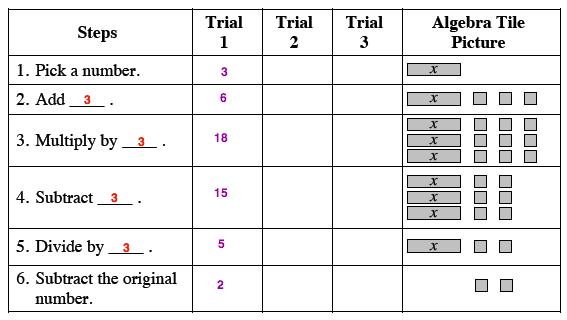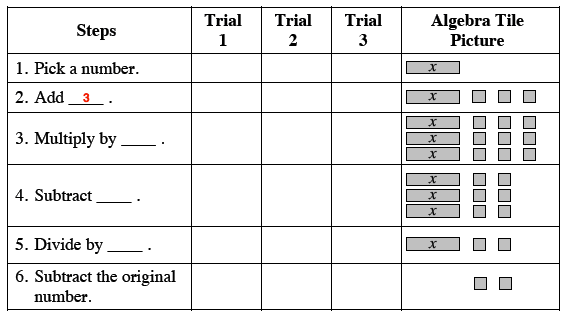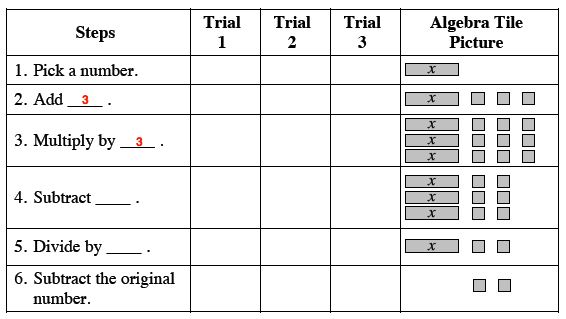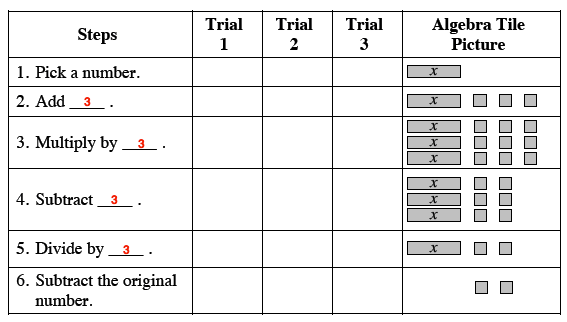### Home > MC2 > Chapter 5 > Lesson 5.1.1 > Problem5-9

5-9.

Draw the table below on your paper and look carefully at the algebra tiles in order to fill in each of the "Steps." Use your own numbers in the trials, again considering fractions, decimals, and integers. Homework Help ✎

Steps

Trial 1

Trial 2

Trial 3

Algebra Tile Picture

1. Pick a number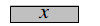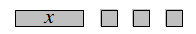3. Multiply by ____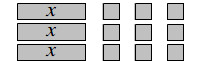4. Subtract ____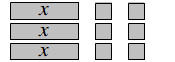5. Divide by ____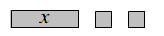6. Subtract the original number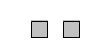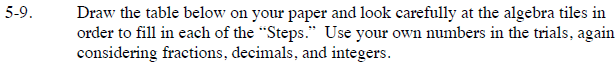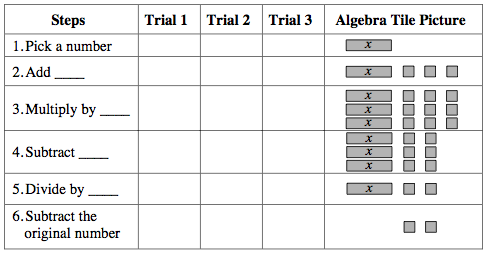Notice how the tiles increase or decrease after each step.

By how much did the Algebra tiles increase in Step 2?

By how much did that number multiply in Step 3?
Now complete the rest of the steps using this method.

Substitute in your own numbers for each trial.